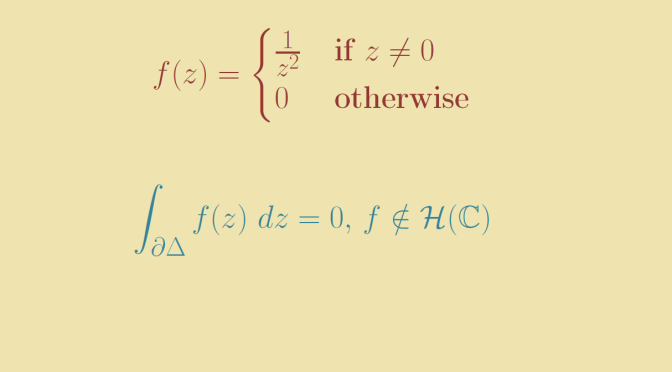# Counterexample around Morera’s theorem

Let’s recall Morera’s theorem.

Morera’s theorem
Suppose that $$f$$ is a continuous complex-valued function in a connected open set $$\Omega \subset \mathbb C$$ such that
$\int_{\partial \Delta} f(z) \ dz = 0$ for every closed triangle $$\Delta \subset \Omega \setminus \{p\}$$ where $$p \in \Omega$$. Then $$f$$ is holomorphic in $$\Omega$$.

Does the conclusion of Morera’s theorem still hold if $$f$$ is supposed to be continuous only in $$\Omega \setminus \{p\}$$? The answer is negative and we provide a counterexample.

Let $$\Omega$$ be the entire complex plane, $$f$$ defined as follows
$f(z)=\begin{cases} \frac{1}{z^2} & \text{if } z \neq 0\\ 0 & \text{otherwise} \end{cases}$ and $$p$$ the origin.

For $$a,b \in \Omega \setminus \{0\}$$ we have
\begin{aligned} \int_{[a,b]} f(z) \ dz &= \int_{[a,b]} \frac{dz}{z^2}\\ &= \int_0^1 \frac{b-a}{[a+t(b-a)]^2} \ dt\\ &=\left[ -\frac{1}{a+t(b-a)} \right]_0^1 = \frac{1}{a} – \frac{1}{b} \end{aligned}

Hence for a triangle $$\Delta$$ with vertices at $$a,b ,c \in \Omega \setminus \{0\}$$:
$\int_{\partial \Delta} f(z) \ dz = \left( \frac{1}{a} – \frac{1}{b} \right) + \left( \frac{1}{b} – \frac{1}{c} \right) + \left( \frac{1}{c} – \frac{1}{a} \right)=0$

However, $$f$$ is not holomorphic in $$\Omega$$ as it is even not continuous at $$0$$.